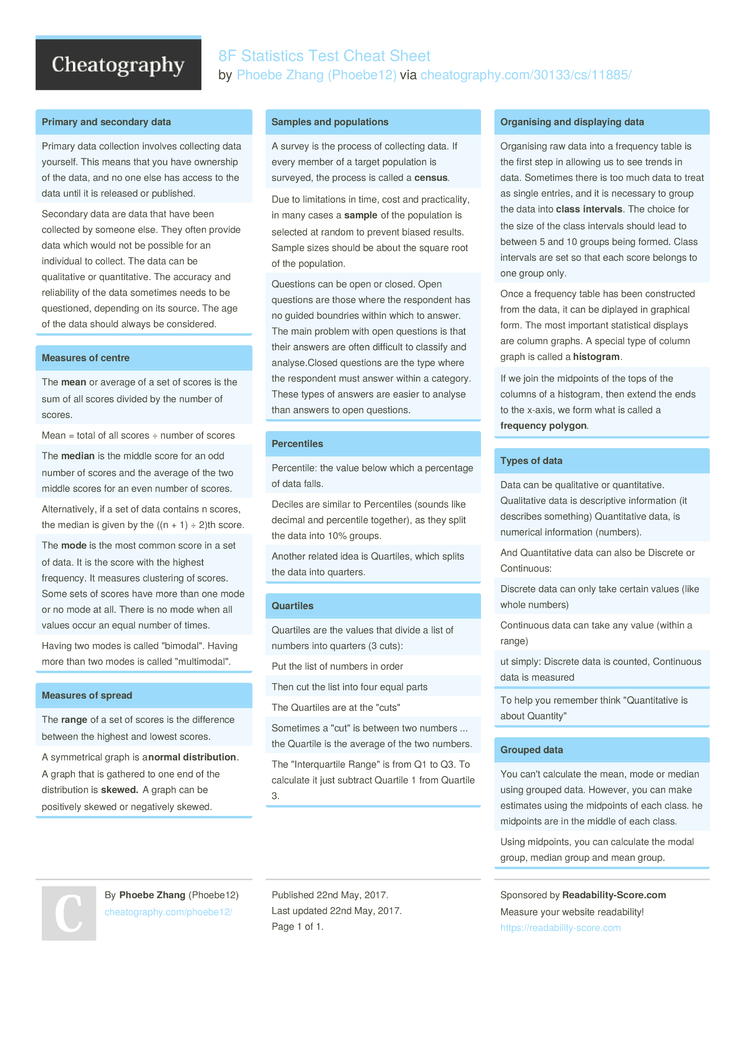# 8F Statistics Test Cheat Sheet by Phoebe12

### Primary and secondary data

 Primary data collection involves collecting data yourself. This means that you have ownership of the data, and no one else has access to the data until it is released or published. Secondary data are data that have been collected by someone else. They often provide data which would not be possible for an individual to collect. The data can be qualit­ative or quanti­tative. The accuracy and reliab­ility of the data sometimes needs to be questi­oned, depending on its source. The age of the data should always be consid­ered.

### Measures of centre

 The mean or average of a set of scores is the sum of all scores divided by the number of scores. Mean = total of all scores ÷ number of scores The median is the middle score for an odd number of scores and the average of the two middle scores for an even number of scores. Altern­ati­vely, if a set of data contains n scores, the median is given by the ((n + 1) ÷ 2)th score. The mode is the most common score in a set of data. It is the score with the highest frequency. It measures clustering of scores. Some sets of scores have more than one mode or no mode at all. There is no mode when all values occur an equal number of times. Having two modes is called "­bim­oda­l". Having more than two modes is called "­mul­tim­oda­l".

 The range of a set of scores is the difference between the highest and lowest scores. A symmet­rical graph is a normal distri­but­ion. A graph that is gathered to one end of the distri­bution is skew­ed. A graph can be positively skewed or negatively skewed.

### Samples and popula­tions

 A survey is the process of collecting data. If every member of a target population is surveyed, the process is called a cens­us. Due to limita­tions in time, cost and practi­cality, in many cases a sample of the population is selected at random to prevent biased results. Sample sizes should be about the square root of the popula­tion. Questions can be open or closed. Open questions are those where the respondent has no guided boundries within which to answer. The main problem with open questions is that their answers are often difficult to classify and analys­e.C­losed questions are the type where the respondent must answer within a category. These types of answers are easier to analyse than answers to open questions.

### Percen­tiles

 Percen­tile: the value below which a percentage of data falls. Deciles are similar to Percen­tiles (sounds like decimal and percentile together), as they split the data into 10% groups. Another related idea is Quartiles, which splits the data into quarters.

### Quartiles

 Quartiles are the values that divide a list of numbers into quarters (3 cuts): Put the list of numbers in order Then cut the list into four equal parts The Quartiles are at the "­cut­s" Sometimes a "­cut­" is between two numbers ... the Quartile is the average of the two numbers. The "­Int­erq­uartile Range" is from Q1 to Q3. To calculate it just subtract Quartile 1 from Quartile 3.

### Organising and displaying data

 Organising raw data into a frequency table is the first step in allowing us to see trends in data. Sometimes there is too much data to treat as single entries, and it is necessary to group the data into class interv­als. The choice for the size of the class intervals should lead to between 5 and 10 groups being formed. Class intervals are set so that each score belongs to one group only. Once a frequency table has been constr­ucted from the data, it can be diplayed in graphical form. The most important statis­tical displays are column graphs. A special type of column graph is called a hist­ogr­am. If we join the midpoints of the tops of the columns of a histogram, then extend the ends to the x-axis, we form what is called a freq­uency polygon.

### Types of data

 Data can be qualit­ative or quanti­tative. Qualit­ative data is descri­ptive inform­ation (it describes something) Quanti­tative data, is numerical inform­ation (numbers). And Quanti­tative data can also be Discrete or Contin­uous: Discrete data can only take certain values (like whole numbers) Continuous data can take any value (within a range) ut simply: Discrete data is counted, Continuous data is measured

### Grouped data

 You can't calculate the mean, mode or median using grouped data. However, you can make estimates using the midpoints of each class. he midpoints are in the middle of each class. Using midpoints, you can calculate the modal group, median group and mean group.1 Page
//media.cheatography.com/storage/thumb/phoebe12_8f-statistics-test.750.jpg

PDF (recommended)